Home > A2C > Chapter 2 > Lesson 2.1.7 > Problem2-105

2-105.
1. Describe the domain of each function or sequence below. 2-105 HW eTool (Desmos). Homework Help ✎

1. The function f(x) = 3x − 5.

2. The sequence t(n) = 3n − 5.

3. The function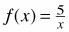.

4. The sequence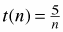.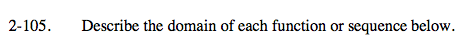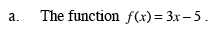The domain is any value of x that yields a real number value for y.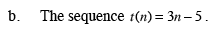Keep in mind that this is a sequence, not a function.

1, 2, 3...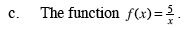Can 5 be divided by any number?

x cannot equal zero.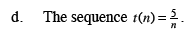Keep in mind that this is a sequence, not a function.

Use the eTool below to explore the domains.
Click the link at right for the full version of the eTool: A2C 2-105 HW eTool